# Finding Percentage Increase and Decrease

There are 10 children in each group below.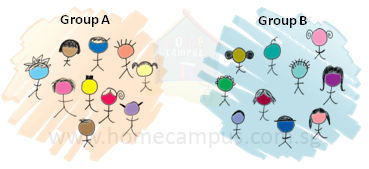2 more children join Group A.

The number of children in Group A increases by 2.

 Percentage increase = 210 × 100% = 20%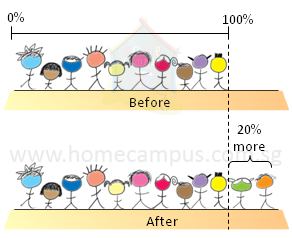There are now 20% more children in Group A than before.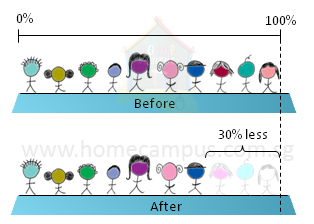3 children from Group B leave the group.

The number of children in Group B decreases by 3.

 Percentage decrease = 310 × 100% = 30%
There are now 30% fewer children in Group B than before.

 Percentage increase = amount increasedinitial quantity × 100%

 Percentage decrease = amount decreasedinitial quantity × 100%

### Word Problem 1

Lisa's salary was raised from \$4000 to \$4800 per month. What was the percentage increase in her monthly salary?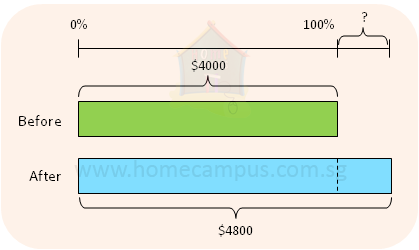Percentage increase = \$800\$4000 × 100% = 20%

The percentage increase in her monthly salary was 20%.

### Word Problem 2

The price of a chair was reduced from \$270 to \$189 in a sale. Find the percentage discount on the chair.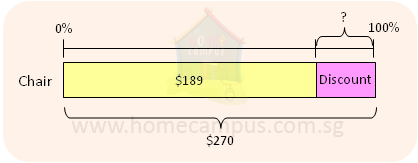Decrease in price = \$270 − \$189 = \$81

 Percentage decrease = \$81\$270 × 100% = 30%

The percentage discount on the chair was 30%.

### Word Problem 3

Joey had a leaky water bottle that leaked 15 ml of water every 10 minutes. What was the percentage decrease in the water in the bottle 1 hour after the bottle was filled up to 450 ml?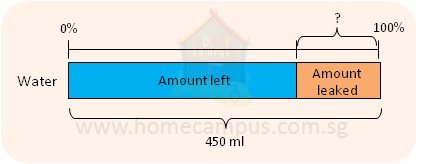10 minutes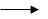15 ml 1 minute1510 ml 60 minutes1510 ml × 60 = 90 ml
After 1 hour 90 ml of water had leaked from the bottle.

 Percentage decrease = 90450 × 100% = 20%

The percentage decrease in the water after 1 hour was 20%.

### Word Problem 4

Due to some roadworks on the usual route from home to school, Peggy has to take another route to school which is 7% longer. This increase in distance is 126 m. What is the length of the new route?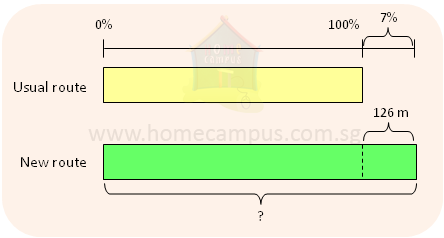7%126 m 1%1267 m = 18 m 107%18 m × 107 = 1926 m

The length of the new route is 1926 m.

### Word Problem 5

Sue bought a piece of cloth 140 cm long. After washing it, the cloth shrank by 4% along its length. What is the length of the cloth now?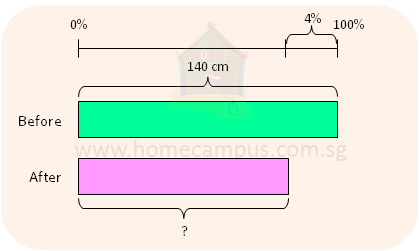100% − 4% = 96% 96% of 140 cm = 96100 × 140 cm = 134.4 cm

The length of the cloth is 134.4 cm now.